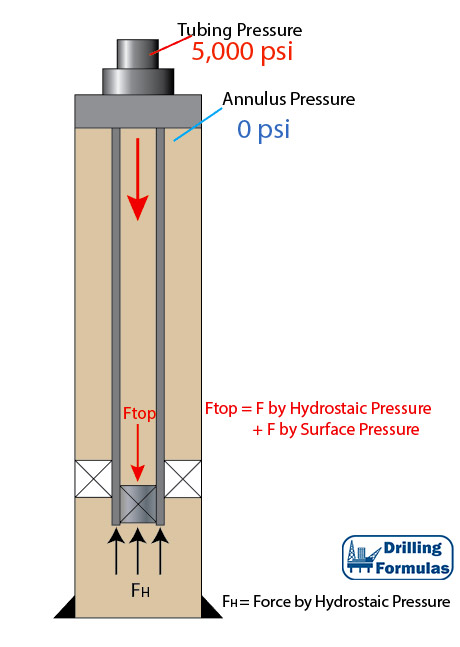## Piston Force on Closed-Ended Tubular (Plugged Tubular)According to the previous post (piston force on open-ended tubular), applied surface pressure will reduce tensile force on surface. In this article, this is an analysis on the piston force on a plugged tubular string and the details are shown below;

Tubing Detail

• 5” Tubing
• ID of tubing = 3.696”
• Packer seal bore OD = 5.25”
• Weight per length = 17.7 lb/ft
• Total Length = 10,000 ft
• Plugged tubing depth = 10,000 ft
• Fluid density = 10 ppg
• Tubing is free to move in the packer
• Applied surface pressure = 5,000 psi

Figure 1 shows the wellbore schematic. Applied pressure (5,000 psi) will cause a piston effect to push the tubing. Therefore, at the bottom of tubing buoyancy and piston force will act in an upwards direction (compression).Figure 1 – Wellbore Schematic